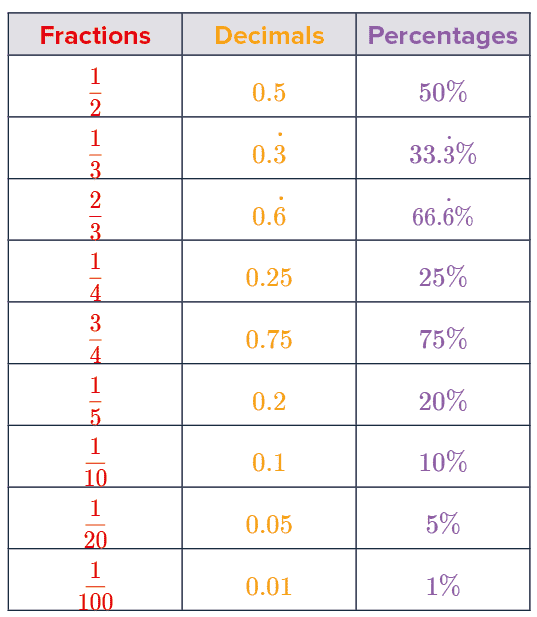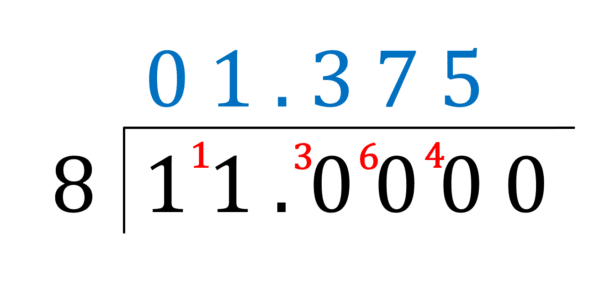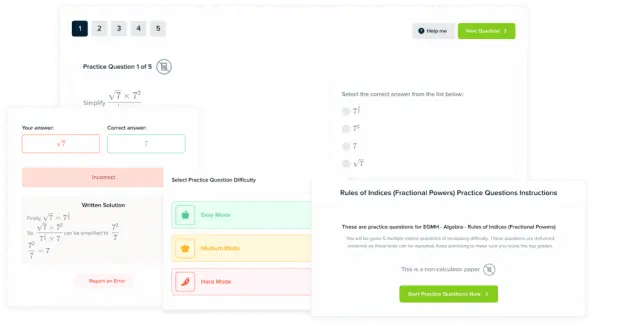# Fractions Decimals and Percentages

GCSEKS3Level 1-3AQACambridge iGCSEEdexcelEdexcel iGCSEOCRWJEC

## Fractions, Decimals, and Percentages

Fractions, decimals and percentages are just different ways of expressing a proportion of a value. Each is equally valid and the choice of which is used is down to the context and/or setting it is being used in.

Here we will explain how to convert between fractions, decimals, and percentages (in both directions).

## Common Conversions

In shops, you will frequently see discounts written as $50\%$ off or $\dfrac{1}{2}$ price but never as $0.5$ times the original cost. Similarly when converting currency, the exchange rate will never be be quoted as a percentage but rather as a decimal.

All three are mathematically equivalent ways of saying the same thing but we default to using the one that is most appropriate in that scenario.

The table shows some important common conversions between fractions, decimals and percentages that you should remember.Level 1-3GCSEKS3AQAEdexcelOCRWJECCambridge iGCSEEdexcel iGCSE
Level 1-3GCSEKS3AQAEdexcelOCRWJECCambridge iGCSEEdexcel iGCSE

## Fractions to Decimals to Percentages

Convert fractions to decimals – treat the fraction like a division and divide the number on the top by the number on the bottom. e.g. $\dfrac{9}{20} = 9 \div 20 = 0.45$

Convert decimals to percentages – multiply by $100$ (move the decimal point right $2$ places). e.g.  $0.45 \times 100 = 45\%$Level 1-3GCSEKS3AQAEdexcelOCRWJECCambridge iGCSEEdexcel iGCSE

## Percentages to Decimals to Fractions

Convert percentages to decimals – divide by $100$ (shift the decimal point left $2$ places). e.g. $64 \% \div 100 = 0.64$

Convert decimals to fractions – for terminating decimals (i.e. decimals that don’t go on forever) we write the decimal as a fraction with $1$ on the bottom. Then, keep multiplying top and bottom by $10$ until the decimal becomes a whole number. Then you can simplify the fraction if needed.

e.g. $0.79 = \dfrac{0.79}{1} = \dfrac{7.9}{10} = \dfrac{79}{100}$

For recurring decimals it can be more tricky (see the recurring decimals to fractions page)Level 1-3GCSEKS3AQAEdexcelOCRWJECCambridge iGCSEEdexcel iGCSE
Level 1-3GCSEKS3AQAEdexcelOCRWJECCambridge iGCSEEdexcel iGCSE

## Example 1: Percent and Decimal Conversions

a) Write $37\%$ as a decimal.

[1 mark]

To convert this to a decimal we will divide by $100$, so we get $37 \div 100 = 0.37$

b) Write $0.548$ as a percentage.

[1 mark]

To convert this to a percentage we will multiply by $100$, so we get $0.548 \times 100 = 54.8\%$

Level 1-3GCSEKS3AQAEdexcelOCRWJECCambridge iGCSEEdexcel iGCSE

## Example 2: Fraction to Decimal

a) Write $\dfrac{12}{25}$ as a decimal.

[2 marks]

Dividing $12$ by $25$ doesn’t sound too pleasant, but there is a way we can change the fraction (before dividing top by bottom) to make life easier. Notice that $25\times 4=100$ and dividing by $100$ is straightforward. So, if we multiply top and bottom by $4$ we get

$\dfrac{12}{25} \times \dfrac{4}{4} =\dfrac{48}{100}=0.48$

b) Write $\dfrac{11}{8}$ as a decimal.

[2 marks]

In this case, there is no nice shortcut. We’ll just have to divide $11$ by $8$ in whichever way feels most comfortable – here, we’ll go for the bus stop method.Doing this, we get the picture shown above, (click here to revise the bus stop method) and we see that the result is,

$\dfrac{11}{8} = 1.375$

Level 1-3GCSEKS3AQAEdexcelOCRWJECCambridge iGCSEEdexcel iGCSE
Level 1-3GCSEKS3AQAEdexcelOCRWJECCambridge iGCSEEdexcel iGCSE

## Example 3: Decimal to Fraction

Write $4.56$ as a fraction in its simplest form.

[2 marks]

Any number divided by $1$ is equal to itself, so we can write $4.56$ as $\dfrac{4.56}{1}$.

Now, if we multiply top and bottom by $100$ we get

$\dfrac{4.56}{1}=\dfrac{4.56\times 100}{1\times 100}=\dfrac{456}{100}$

All that remains now is to simplify it. Cancelling down,

$\dfrac{456}{100} = \dfrac{228}{50} = \dfrac{114}{25}$

Level 1-3GCSEKS3AQAEdexcelOCRWJECCambridge iGCSEEdexcel iGCSE

## Example 4: Percentage to Fraction

Write $48.1\%$ as a fraction.

[1 mark]

Percentages are already out of $100$, so

$48.1\%=\dfrac{48.1}{100}$

Then, multiplying top and bottom by $10$ (to make the numbers whole) we get

$48.1\% = \dfrac{481}{1000}$

Level 1-3GCSEKS3AQAEdexcelOCRWJECCambridge iGCSEEdexcel iGCSE

## Example 5: Fraction to Percentage

Write $\dfrac{4}{5}$ as a percentage.

[1 mark]

Firstly, let’s convert it to a decimal. Notice that if we times top and bottom by $2$, the fraction becomes

$\dfrac{4}{5}=\dfrac{4\times 2}{5\times 2} = \dfrac{8}{10} = 8\div 10 = 0.8$

Then, to convert this decimal to a percentage, we times by $100$:

$0.8 \times 100 = 80\%$

Note: Alternatively, if you can write a fraction with $100$ on the denominator, then the value on the top immediately gives you what the fraction would be as a percentage. For example, $\dfrac{34}{50}=\dfrac{68}{100}=68\%$

Level 1-3GCSEKS3AQAEdexcelOCRWJECCambridge iGCSEEdexcel iGCSE

## Fractions Decimals and Percentages Example Questions

We can express this percentage as a decimal simply by dividing by $100$,

$55.4\%\div100=0.544$Gold Standard Education

We can express this percentage as a fraction by dividing by $100$,

$\dfrac{16.4}{100}$

To make the numbers whole, we multiply top and bottom by $10$,

$\dfrac{16.4\times 10}{100 \times 10}=\dfrac{164}{1,000}$

Now, we simplify until there are no common factors left. So, we find,

$\dfrac{164}{1,000}=\dfrac{82}{500}=\dfrac{41}{250}$Gold Standard Education

We will treat the fraction like a division and divide $17$ by $40$,

$\;\;\;\;\;0\;0.\;\;4\;\;\;2\;\;\;5\\40\overline{\left)1{}^17.{}^{17}0{}^{10}0{}^{20}0\right.}$

Therefore,

$\dfrac{17}{40}=0.425$Gold Standard Education

We can express this decimal as a fraction,

$0.256=\dfrac{0.256}{1}$

To make the numbers whole, we multiply top and bottom by $1000$,

$\dfrac{0.256}{1}\times \dfrac{1000}{1000}=\dfrac{256}{1000}$

Now, we simplify until there are no common factors left. So, we find,

$\dfrac{256}{1000}=\dfrac{32}{125}$Gold Standard Education

A simpler method than diving $13$ by $20$ in its current form is to notice that $20 \times 5 = 100$ and dividing by $100$ is straightforward. So, if we multiply top and bottom by $5$ we get,

$\dfrac{13}{20}\times\dfrac{5}{5}=\dfrac{65}{100} = 0.65$Gold Standard Education

## Fractions Decimals and Percentages Worksheet and Example Questions

### (NEW) Fractions to Decimals to Percentages Exam Style Questions - MME

Level 1-3GCSEProduct